# Understanding cavitation

• I
• JD_PM
JD_PM
Cavitation is a phenomena that occurs in liquids when the local static pressure drops below the vapor pressure; bubbles form within the liquid.

I am studying cavitation in incompressible, two-phase (liquid & gas), homogeneous mixture of cryogenic fluids (liquid nitrogen in particular) within pipes and would like to understand the thermal effects involved. My main sources are: 1) "Implementation and validation of an extended Schnerr-Sauer cavitation model for non-isothermal flows in OpenFOAM" (section 2.3: Thermal model, picture below; it's open source so no issues) and 2) "Method for prediction of pump cavitation performance for various liquids, liquid temperatures, and rotative speeds" (section: Determination of Cavity Pressure Depressions, picture below; it's open source so no issues).

I would like to understand and discuss the thermal models they propose and see if they are applicable to my particular case.

When referring to heat transfer I mean convective heat transfer (which I assume to be a combination of conduction and advection).

1) They state "The convective heat transfer term is subtracted from the latent heat source term". Why? What I understand from this is that latent heat and heat transfer compete but I do not quite get why this should be the case. Let's look at the case of vaporization; the vaporization heat needed for vaporization of a liquid is the convective heat transfer needed to reach vaporization temperature and the latent heat of vaporization of the liquid, and not the difference...

2) They set a heat balance between the heat required for vaporization and the heat drawn from the liquid surrounding the cavity (that is, the liquid-vapor interface).

So I think that they are essentially stating the same idea: the net heat transfer for vaporization is the difference between net latent heat transfer and convective heat transfer.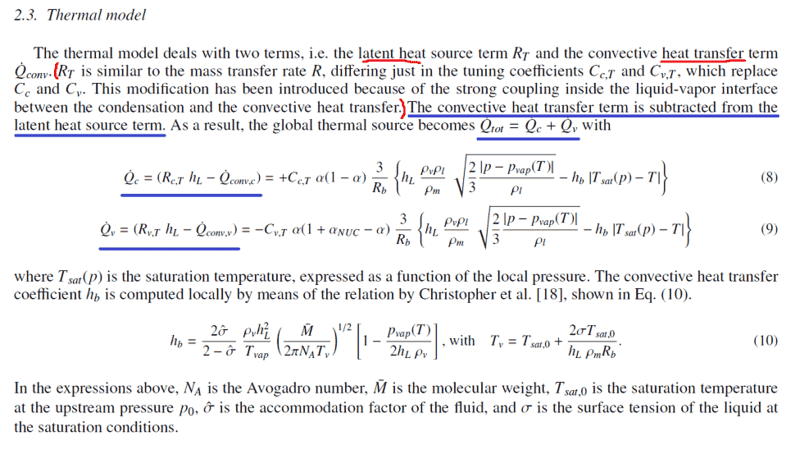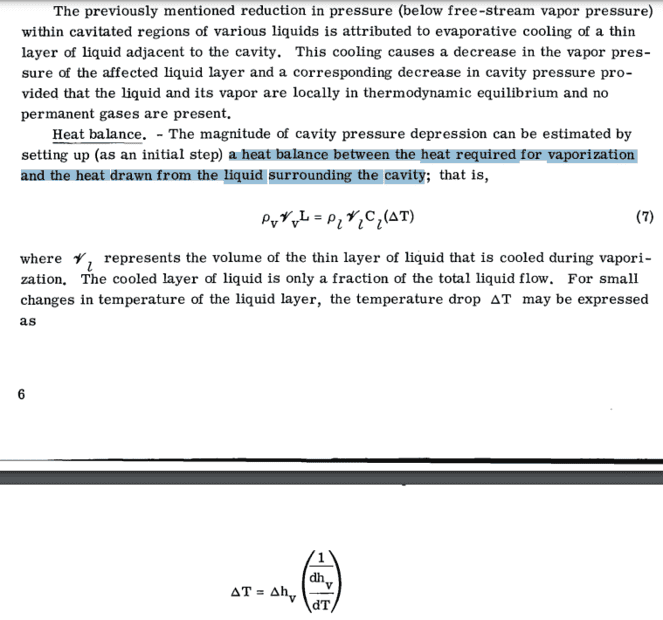JD_PM
Let me go more to the point.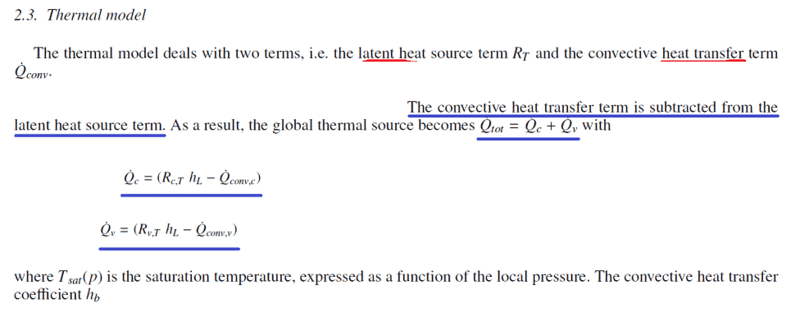I would like to understand why the convective heat transfer term is subtracted from the latent heat source term to obtain the total heat transfer.

Message to anyone reading: even though you have never dealt with cavitation your input/guess would be useful. Discussion would definitely be much appreciatedPlease let me know if further clarifications are needed.

Hello Sr. @Chestermiller, you might find the topic interesting.

Mentor
Have you tried contacting the authors?

JD_PM
Hi DrClaude

I understand it's a bit too much to read a paper, my text and then reply.

I am planning to post a thread that doesn't require to read any paper beforehand.

•vanhees71
Mentor
I did look at the paper, but this would be a case of "your guess is as good as mine." And your guess is probably betterThe don't seem to give the values of ##R_{c,T}## or ##R_{v,T}##, which would have been helpful (I would like to know the sign).

•vanhees71 and JD_PM
Arjan82
So, here is a guess (I haven't read it too carefully though):

What they are trying to achieve is a heat source term for the cavity (correct?). This will influence its temperature and pressure, naturally. So, the convective term is the term (apparently) that removes heat away from the cavity. The latent heat term is the source of heat due to condensation (negative I guess for vaporization). So the difference between the two would then be the heat source that influences the pressure and temperature of the cavity.

•JD_PM
JD_PM
I did look at the paper, but this would be a case of "your guess is as good as mine." And your guess is probably betterNo worries, your above reply is appreciatedThe don't seem to give the values of ##R_{c,T}## or ##R_{v,T}##, which would have been helpful (I would like to know the sign).

They do, indirectly though. ##R_{c,T}## and ##R_{v,T}## are latent heat source terms. In the paper, they assume they have exactly the same form than the mass transfer Schnerr-Sauer source terms (that is, the coefficients that rule how much mass is transferred from liquid to vapor and vice versa):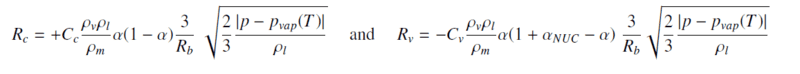They just changed ##C_c## and ##C_v## for ##C_{c,T}## and ##C_{v,T}##

Let's not discuss how to derive the source terms at the moment (for that we'd need to start discussing about the Rayleigh-Plesset equation).

What I think is interesting to do is discussing why the mass transfer can be modeled the same way than latent heat. They justify it due to the "strong coupling inside the liquid-vapor interface between the condensation and the convective heat transfer."

I think what they mean is that the energy lost by the vapor and transferred to the liquid by means of latent heat of condensation is linked to the energy lost by the liquid and gained by the vapor by means of convective heat transfer.•vanhees71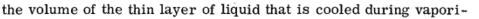•